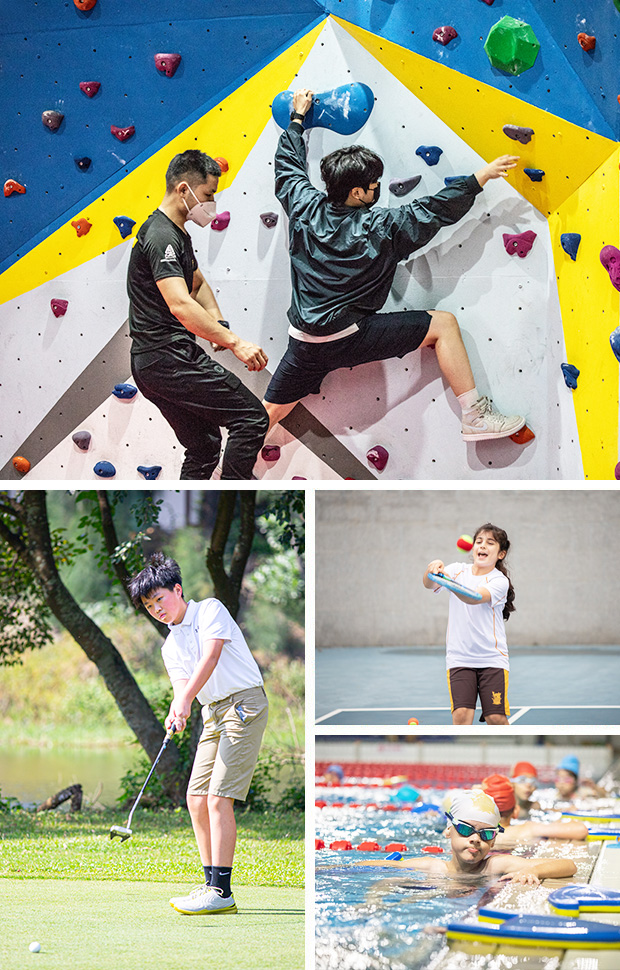• 视觉艺术课
• 篮球
• 足球
• 跳绳
• 体能
• 中国舞蹈
• 街舞
• 拉丁舞
• 奥尔夫乐器
• 戏剧课
• 游泳
• 小提琴
• 钢琴
• 架子鼓
• 烘焙
• KMI逻辑训练
• 乐高机器人

• 乐高俱乐部
• 图书馆读书会
• 趣味数学
• 足球训练
• 西式烘焙
• 手工创作
• 足球
• 滑板训练
• 毛笔书法
• 超级演说
• 音乐故事与乐理
• 羽毛球训练
• 中国文化艺术赏析
• 奥数课
• 琵琶
• 网球训练
• 合唱
• 舞蹈
• 国际象棋
• 机器人乐高课
• 古筝
• 尤克里里
• 硬笔书法
• 礼仪和国学课
• 手机摄影
• 西班牙语 初级
• 篮球
• 游泳
• 棋盘游戏
• 陶笛俱乐部
• 高尔夫
• 主持人
• 滑板俱乐部
• 教育版我的世界
• 综合运动
• 围棋

• 桌球
• 剪纸
• 语言实验室
• 象棋
• 纪录片
• 游泳
• 乒乓球
• 世界学者杯训练
• 乐理
• 粤语
• 碰式橄榄球
• 篮球训练
• 足球
• 体能训练
• 健身
• 中国文化
• 游戏编程
• 网球
• 桌游
• 舞蹈
• 流行歌手学生俱乐部
• 高中排球
• 经典名著的读写
• 滑板
• 3D制作俱乐部
• 自然地理鉴赏
• 冥想
• 高中数学竞赛
• 美国大学探究学生俱乐部
• 羽毛球训练
• 初中奥数
• 脱口秀
• 英语配音和角色扮演
• 钩针编织
• 游戏理论学生俱乐部
• 机器人乐高
• 羽毛球
• 电影赏析
• 钢琴表演交流
• 心理学
• 设计&制作
(限九十年级)
• 高尔夫
• 舞蹈综合
• 我的世界
• 社会公正问题研究学生俱乐部
• 模拟联合国
• 英语听力俱乐部
• 壁球
• 高中飞行模拟器
• 工程技术挑战
(限八年级)
• 哲学学生俱乐部
• 篮球
• 意大利语
* 中学部和双语中学部学生可根据求学需求视实际情况实现校内互转。